How to Make a Control Chart with Excel VBA!

In this article, I will show you How to Make a Control Chart with Excel VBA. Control charts are widely used to monitor process stability and control.

The process data are always plotted in order with three lines added:

1. A central line for the average,
2. An upper line for the upper control limit,
3. And a lower line for the lower control limit.

If a process is stable and in control, the process data will fall within control limits. Otherwise, the data will fall out of control limits. By comparing process data against these lines, we can draw conclusions about whether the process variation is in control or is out of control.

Based on above description, we can see that a control chart can be developed by following 4 steps:

• Draw a series graph
• Add a central line which is a reference line to indicate process location
• Add the other reference lines – upper and lower lines – to show process dispersion
• Customize the chart to make it more beautiful

Let’s create the dummy data

Before diving into programming world, let’s use Excel RND function to create random dummy data which will be used later to plot control chart. Suppose that the random number represents 20 high school students’ height and will fall between 158 and 180. By running following code, we can get 20 random numbers.

 Source code```Sub DummyData()
Worksheets(1).Cells(1, 1) = "Student No"
Worksheets(1).Cells(1, 2) = "Height"
'Apply RND function to create random dummy data
For i = 2 To 21
Worksheets(1).Cells(i, 1) = i - 1
Worksheets(1).Cells(i, 2) = Int((180 - 158 + 1) * Rnd + 158)
Next i
End Sub```

With the sample data that we just created, we can use below code to compute mean, LCL and UCL of sample data which will be used to draw central line, lower line and upper line, respectively. We use formula to compute statistics so that values of mean, standard deviation, LCL and UCL can change automatically once we run DummyData to change sample data.

 Source code```'Get last used row in column B
nlast = Worksheets(1).Cells(Rows.Count, "B").End(xlUp).Row
'Compute Mean
For i = 2 To nlast
Cells(i, 3).Formula = "=Average(" & "B2:B" & nlast & ")"
Next i
'Std
For i = 2 To nlast
Cells(i, 4).Formula = "=StDev(" & "B2:B" & nlast & ")"
Next i
'UCL and LCL
For i = 2 To nlast
'UCL
Cells(i, 5).Formula = "=Average(" & "B2:B" & nlast & ") + StDev(" & "B2:B" & nlast & ")*3"
'LCL
Cells(i, 6).Formula = "=Average(" & "B2:B" & nlast & ") - StDev(" & "B2:B" & nlast & ")*3"
Next i
Worksheets(1).Cells(1, 3) = "Mean"
Worksheets(1).Cells(1, 4) = "Std"
Worksheets(1).Cells(1, 5) = "UCL"
Worksheets(1).Cells(1, 6) = "LCL"```

Here’s our dummy data

Here shows how the data looks like and data may vary from time to time when running above code.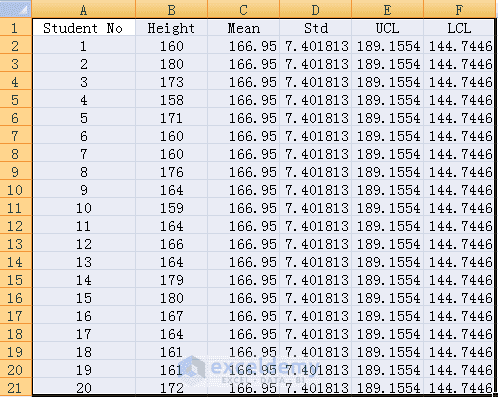Dummy data created by the above code

So far, we have all data essential for control charts and now let’s move on to the most important part – how to draw control charts using VBA programming.

First of all, we need to declare a ChartObject object. The ChartObject object acts as a container for all the elements of a chart. Let’s call it myChtobj but you can use any name. Here display the methods (together with examples) that we will use to manipulate myChtobj object.

 chartobjects.Add(Left, Top, Width, Height) [Create a blank, embedded chart on a worksheet or a chart sheet] Argument Left: The distance between the left edge of the sheet and the right edge of the chart in points Top: The distance between the top of the sheet and the top of the chart in points Width: The width of the chart in points Height: The height of the chart in points Chartobjects(Index) [Refer to a single embedded chart or a collection of all the embedded charts] Argument Index: The name or number of the chart. This argument can be an array, to specify more than one chart Chartobjects(Index).HasTitle = True [Add a title to embedded chart] Chartobjects(Index).ChartTitle.Text = “Height of 20 students” [Set or change title of the embedded chart] Chartobjects(Index).SeriesCollection.Add  source:=Worksheets(“Sheet1”).Range(“B2:B21”) [Add a new series in embedded chart] Chartobjects(Index). ChartType = xlLineMarkers [Specifies the chart type. Option – xlLineMarkers – represents a line with data markers and is suitable for control charts. ]

Creating the control chart

Now let’s try to create elements such as the series graph, central lines, UCL and LCL lines and put them into the chart container. Chart.SeriesCollection.NewSeries method is available for use. Following shows how to plot series graph.

 Source code```Set MyNewSrs = myChtObj.Chart.SeriesCollection.NewSeries
With MyNewSrs
.Name = "Data"
.Values = Worksheets("Sheet1").Range("B2:B21")
End With```

By repeating adding new series using above approach, we can get a graph like below.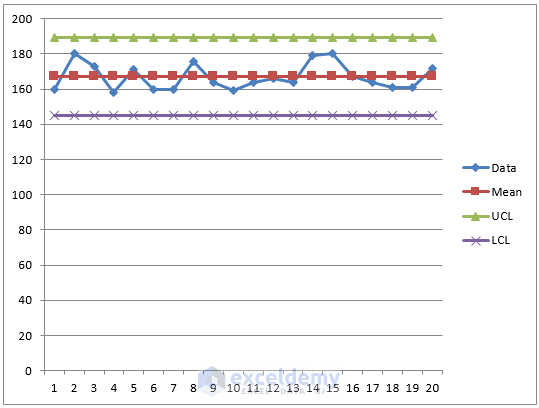However, it looks ugly. First of all, we need to remove data markers from central line, upper line as well as lower line and change the foreground color of the series. Here gives you some methods to change series and markers properties. In order to remove markers, we can just set value of MarkerStyle as xlNone.

 Source code```'Data
Set MyNewSrs = myChtObj.Chart.SeriesCollection.NewSeries
With MyNewSrs
.Name = "Data"
.Values = Worksheets("Sheet1").Range("B2:B21")
.Format.Line.Visible = False
.Format.Line.Visible = True
.Format.Line.ForeColor.RGB = RGB(0, 255, 0)
.Format.Line.Weight = 1
.Format.Line.Transparency = 0
.MarkerSize = 3
.MarkerForegroundColor = RGB(0, 255, 0)
.MarkerBackgroundColor = RGB(0, 255, 0)
.MarkerStyle = xlMarkerStyleCircle
End With```
After formating,we can get following graph.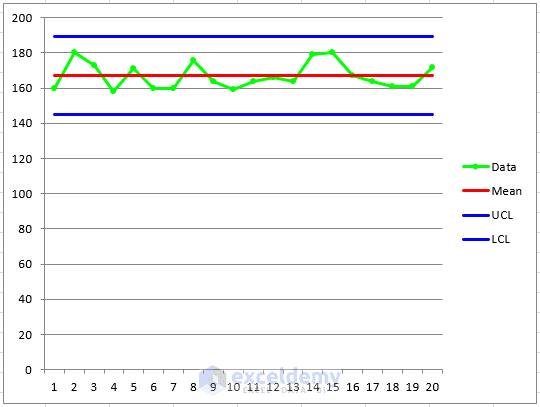Obviously, it is still not beautiful. We need to change legend, axis, and chart itself. There is a little trick when coming to determining y-axis scale. Mod function can be applied to automate the computation of max value and min value (see below in red for details).

Please note that we need to take both source data, UCL and LCL into consideration when trying to compute maximum scale and minimum scale.

 Source code```'Ajust y-axis Scale
'Get max/min among source data, UCL and LCL
Cells(2, 7).Formula = "=max(" & "B2:B" & nlast & ",E2)"
Cells(1, 7) = "Max"
Cells(2, 8).Formula = "=min(" & "B2:B" & nlast & ",F2)"
Cells(1, 8) = "Min"
With myChtObj.Chart.Axes(xlValue, xlPrimary)
.MaximumScale = Int(Cells(2, 7).Value) + (10 - (Int(Cells(2, 7).Value) Mod 10))
.MinimumScale = Int(Cells(2, 8).Value) - (Int(Cells(2, 8).Value) Mod 10)
'Remove major gridlines
.HasMajorGridlines = False
End With```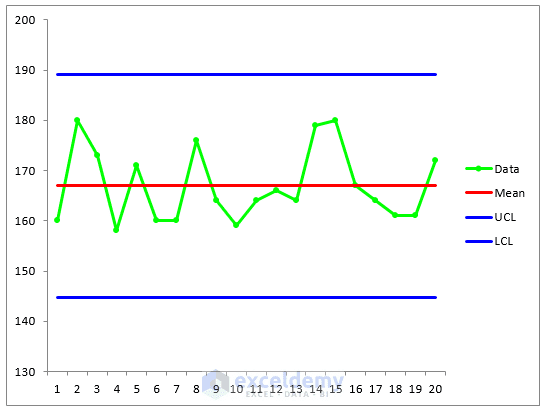And I also present the output above. It looks much better. But I still want to delete legend and insert text next to lines. However, the plot area will become wider than before without legend. Therefore, we need to retrieve the current width of plot area before removing legend and then re-size plot area’s width as before. This can be done by the following code.

 Source code```'Get current width of plot area
pwidth = myChtObj.Chart.PlotArea.Width
'Remove legend
myChtObj.Chart.Legend.Delete
'Set the width of plot area equal to width of orignal one
myChtObj.Chart.PlotArea.Width = pwidth```
But what about inserting text which is near line? We can use the data value label of last marker point to display text what we’d like to show. Here is the code showing you how to change properties of marker point.
 Source code```'Get current width of plot area
pwidth = myChtObj.Chart.PlotArea.Width
'Remove legend
myChtObj.Chart.Legend.Delete
'Set the width of plot area equal to width of orignal one
myChtObj.Chart.PlotArea.Width = pwidth```
Finally, the completed graph is something similar to below. It’s awesome, right?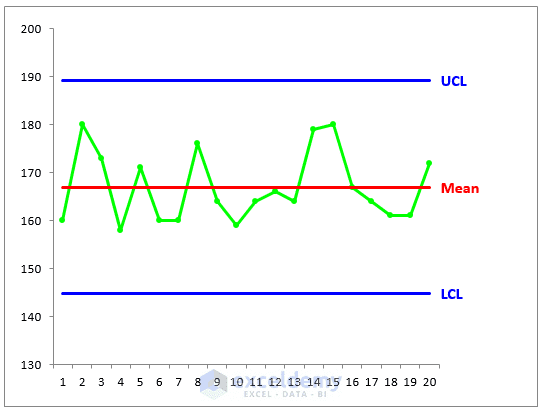Here’s all the code

Here you can find all the code.

 Source code```Sub ControlChart()
'''''''''''''''''''''''''''''''''''''''''''''''''''''''''''''''''''''''''''''
'Part 1 - Calculate '
'''''''''''''''''''''''''''''''''''''''''''''''''''''''''''''''''''''''''''''
'Get last used row in column B
nlast = Worksheets(1).Cells(Rows.Count, "B").End(xlUp).Row
'Compute Mean
For i = 2 To nlast
Cells(i, 3).Formula = "=Average(" & "B2:B" & nlast & ")"
Next i
'Std
For i = 2 To nlast
Cells(i, 4).Formula = "=StDev(" & "B2:B" & nlast & ")"
Next i
'UCL and LCL
For i = 2 To nlast
'UCL
Cells(i, 5).Formula = "=Average(" & "B2:B" & nlast & ") + StDev(" & "B2:B" & nlast & ")*3"
'LCL
Cells(i, 6).Formula = "=Average(" & "B2:B" & nlast & ") - StDev(" & "B2:B" & nlast & ")*3"
Next i
Worksheets(1).Cells(1, 3) = "Mean"
Worksheets(1).Cells(1, 4) = "Std"
Worksheets(1).Cells(1, 5) = "UCL"
Worksheets(1).Cells(1, 6) = "LCL"
'''''''''''''''''''''''''''''''''''''''''''''''''''''''''''''''''''''''''''''
'Part 2 - Chart '
'''''''''''''''''''''''''''''''''''''''''''''''''''''''''''''''''''''''''''''
'Define Object
Dim myChtObj As ChartObject
Set myChtObj = ActiveSheet.ChartObjects.Add(Left:=400, Width:=400, Top:=25, Height:=300)
myChtObj.Chart.ChartType = xlLineMarkers
'Data
Set MyNewSrs = myChtObj.Chart.SeriesCollection.NewSeries
With MyNewSrs
.Name = "Data"
.Values = Worksheets("Sheet1").Range("B2:B21")
.Format.Line.Visible = False
.Format.Line.Visible = True
.Format.Line.ForeColor.RGB = RGB(0, 255, 0)
.Format.Line.Weight = 2
.Format.Line.Transparency = 0
.MarkerSize = 3
.MarkerForegroundColor = RGB(0, 255, 0)
.MarkerBackgroundColor = RGB(0, 255, 0)
.MarkerStyle = xlMarkerStyleCircle
End With
'Central line
Set MyNewSrs = myChtObj.Chart.SeriesCollection.NewSeries
With MyNewSrs
.Name = "Mean"
.Values = Worksheets("Sheet1").Range("C2:C21")
.Format.Line.Visible = False
.Format.Line.Visible = True
.Format.Line.ForeColor.RGB = RGB(255, 0, 0)
.MarkerStyle = xlNone
End With
'Upper line
Set MyNewSrs = myChtObj.Chart.SeriesCollection.NewSeries
With MyNewSrs
.Name = "UCL"
.Values = Worksheets("Sheet1").Range("E2:E21")
.Format.Line.Visible = False
.Format.Line.Visible = True
.Format.Line.ForeColor.RGB = RGB(0, 0, 255)
.MarkerStyle = xlNone
End With
'Lower line
Set MyNewSrs = myChtObj.Chart.SeriesCollection.NewSeries
With MyNewSrs
.Name = "LCL"
.Values = Worksheets("Sheet1").Range("F2:F21")
.Format.Line.Visible = False
.Format.Line.Visible = True
.Format.Line.ForeColor.RGB = RGB(0, 0, 255)
.MarkerStyle = xlNone
End With
'Ajust y-axis Scale
'Get max/min among source data, UCL and LCL
Cells(2, 7).Formula = "=max(" & "B2:B" & nlast & ",E2)"
Cells(1, 7) = "Max"
Cells(2, 8).Formula = "=min(" & "B2:B" & nlast & ",F2)"
Cells(1, 8) = "Min"
With myChtObj.Chart.Axes(xlValue, xlPrimary)
.MaximumScale = Int(Cells(2, 7).Value) + (10 - (Int(Cells(2, 7).Value) Mod 10)) + 10
.MinimumScale = Int(Cells(2, 8).Value) - (Int(Cells(2, 8).Value) Mod 10) - 10
'Remove major gridlines
.HasMajorGridlines = False
End With
'Get current width of plot area
pwidth = myChtObj.Chart.PlotArea.Width
'Remove legend
myChtObj.Chart.Legend.Delete
'Set the width of plot area equal to width of orignal one
myChtObj.Chart.PlotArea.Width = pwidth
'Set marker value label for the last marker
Count = nlast - 1
With myChtObj.Chart.SeriesCollection(2).Points(Count)
.HasDataLabel = Ture
.DataLabel.Characters.Text = Worksheets(1).Cells(1, 3)
.DataLabel.Position = xlLabelPositionRight
.DataLabel.Font.Size = 12
.DataLabel.Font.Bold = True
.DataLabel.Font.Color = RGB(255, 0, 0)
End With
For i = 3 To 4
With myChtObj.Chart.SeriesCollection(i).Points(Count)
.HasDataLabel = Ture
.DataLabel.Characters.Text = Worksheets(1).Cells(1, i + 2)
.DataLabel.Position = xlLabelPositionRight
.DataLabel.Font.Size = 12
.DataLabel.Font.Bold = True
.DataLabel.Font.Color = RGB(0, 0, 255)
End With
Next i
End Sub```

Reminder

Please note that we need to do an examination before starting plotting data because the data should be normally distributed when using control charts. Otherwise, the chart may signal an unexpectedly high rate of false alarms.

How to Create Doughnut, Bubble and Pie of Pie Chart in Excel

How to Make a Pie Chart in Excel (Ultimate Guide)

Zhiping Yan

I am from China and this photo was taken in a classical garden. There are many similar gardens in China, attracting a lot of visitors every year, especially in spring and summer. I was major in Biotechnology. But I took a job as an SAS programmer because I prefer programming. Besides SAS, I also learned Excel VBA in my spare time. It is fantastic to be able to manipulate data, files and even to interact with the internet via programming. This will save me a lot of time. I am keen to learn new things.

Sew Sewram September 6, 2016 at 5:28 PM

Hi
Very interesting, however, control charts are meant for daily data input…the chart should update automatically….can you present one with daily input and automatic chart update?
I use control charts daily and have problems with this, also Mean Std UCL LCL must be in one cell and not in every entry..

Zhiping September 7, 2016 at 7:14 AM

Hi Sew. Thanks for your suggestions. I will write another post later when I am free.